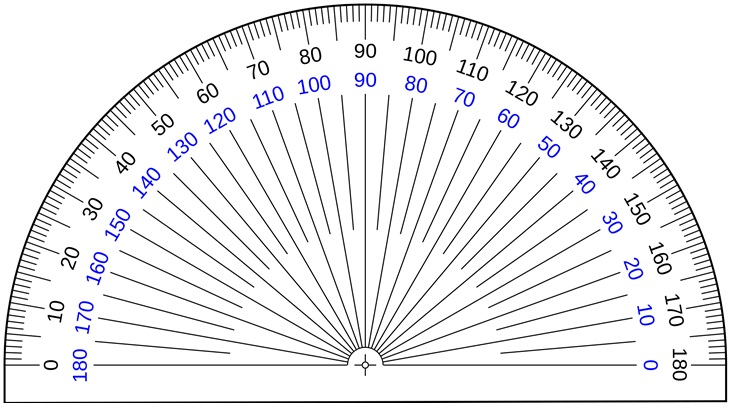To measure any angle,

We use protector.

It is also called a ‘D’

### How to use a protractor?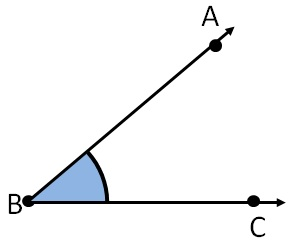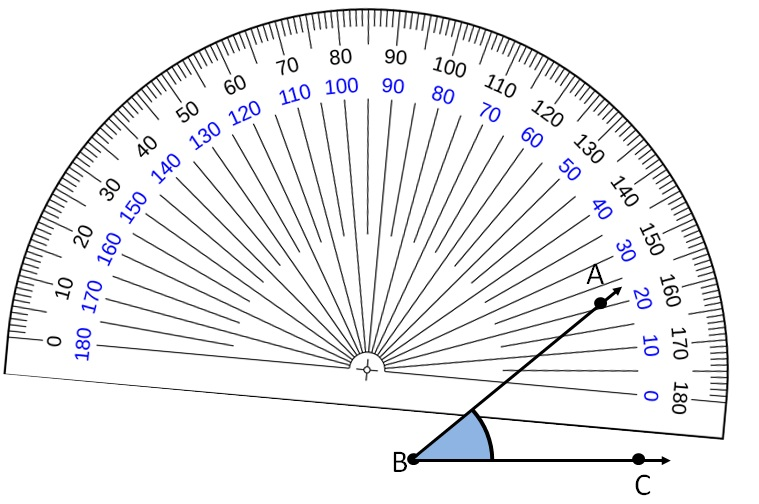So, ∠ABC = 40°

### How to use a protractor?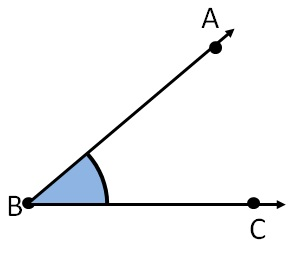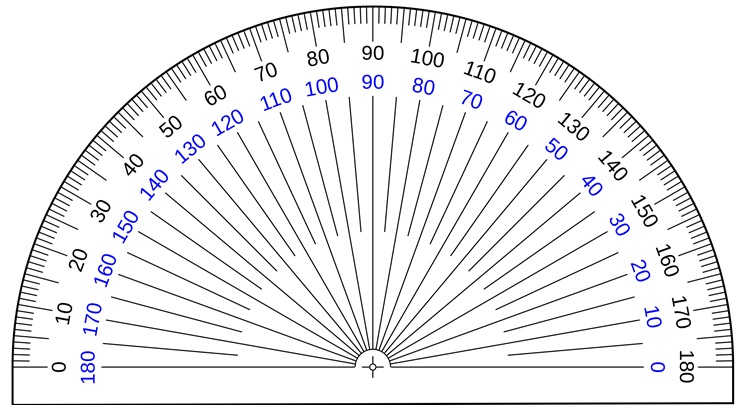So, ∠ABC = 40°

### Measure these angles with a protractor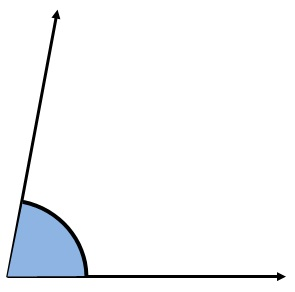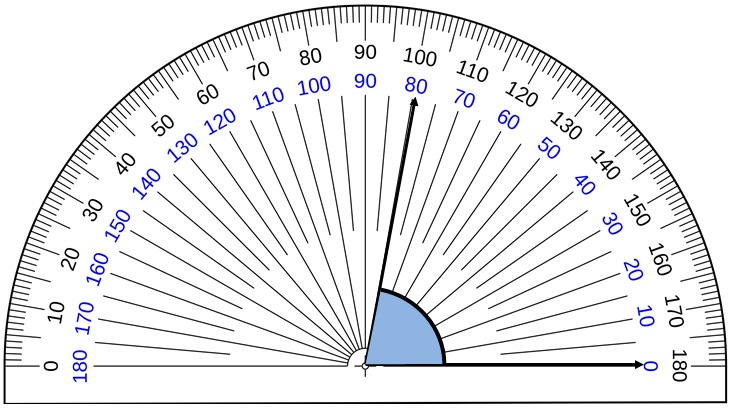So, ∠1 = 80°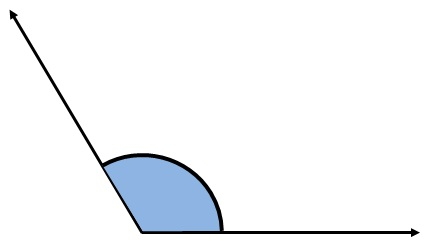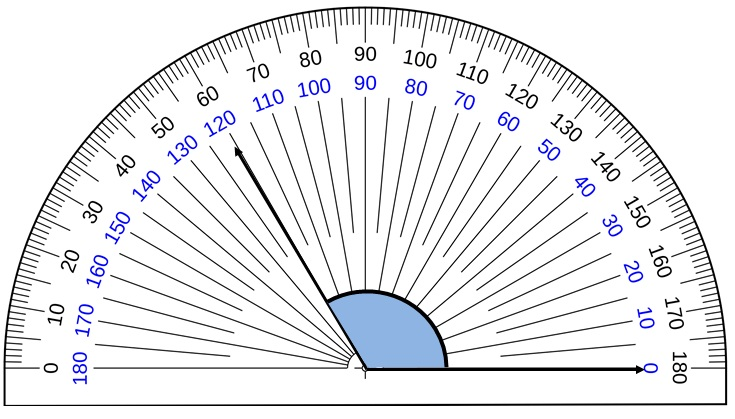So, ∠2 = 120°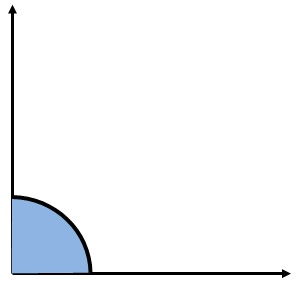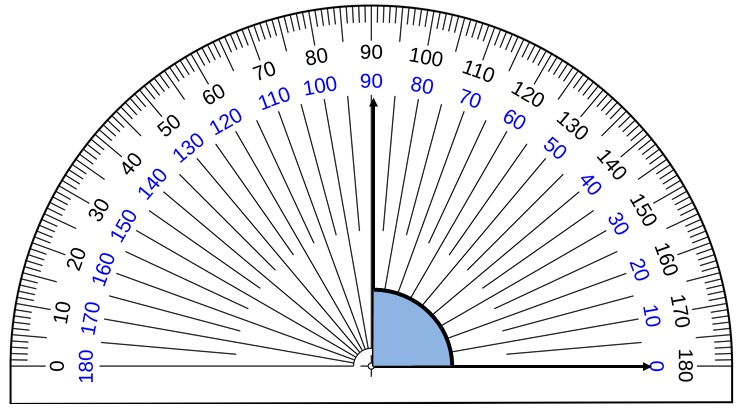So, ∠3 = 90°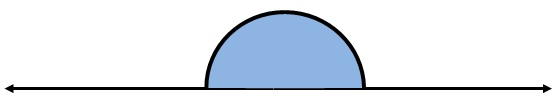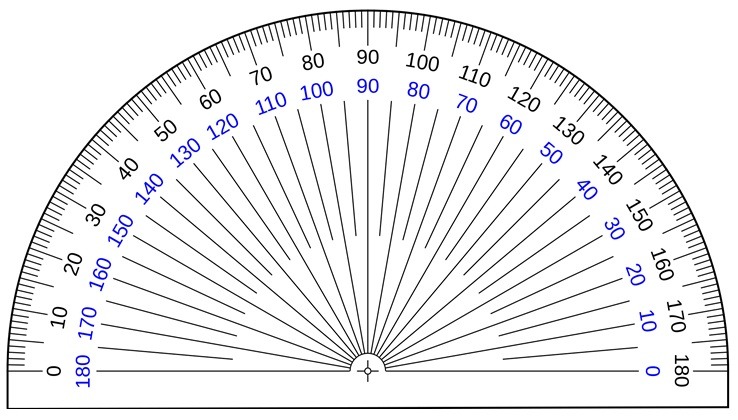So, ∠4 = 180°

1. Chapter 5 Class 6 Understanding Elementary Shapes
2. Concept wise
3. Measuring angles using protractor

Measuring angles using protractor